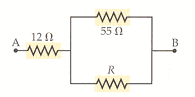# Circuit Resistance Problem

whoopie88
Circuit Resistance Problem!!

## Homework Statement

The equivalent resistance between points A and B of the resistors shown in the figure below is 40 . Find the value of resistance R.## Homework Equations

All circuit formulas.

## The Attempt at a Solution

40 - 12 = 28
28 = [(1/55) + (1/R)]^-1
R = -27

Help!